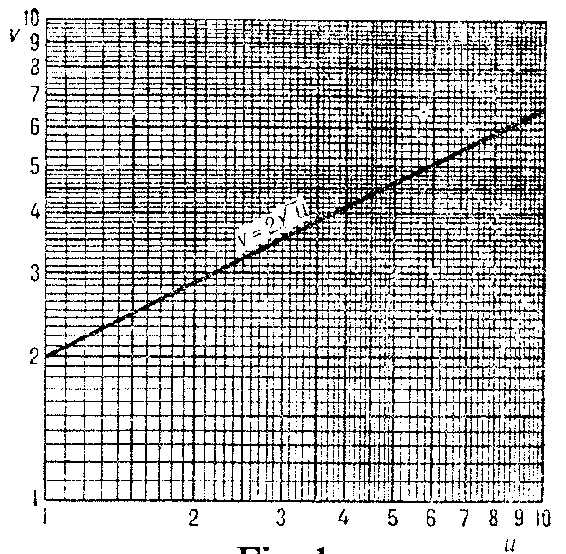# Logarithmic paper

double logarithmic paper

A special form of ruled paper; it is usually made typographically (Fig. a) as follows: On each of the axes of a rectangular $(u,v)$-coordinate system one marks the decimal logarithms of numbers $u$ (on the horizontal axis) and $v$ (on the vertical axis) (cf. also Logarithm of a number); then lines are drawn through the resulting points $(u,v)$ parallel to the axes.Figure: l060620a

There is also semi-logarithmic paper (single logarithmic paper) (Fig. b): On one of the axes of a rectangular $(u,v)$-coordinate system the values of numbers $u$ are marked, and on the other the decimal logarithms of numbers $v$.Figure: l060620b

Logarithmic paper and semi-logarithmic paper are used for drawing the graphs of functions which (in those coordinates) may take a simpler and more obvious form, and in many cases are straight lines. On logarithmic paper straight lines represent functions given by equations of the form $v=au^b$, $u>0$, where $a>0$ and $b$ are constant coefficients; on semi-logarithmic paper straight lines represent functions given by equations of the form $v=ab^u$.

How to Cite This Entry:
Logarithmic paper. Encyclopedia of Mathematics. URL: http://www.encyclopediaofmath.org/index.php?title=Logarithmic_paper&oldid=32138
This article was adapted from an original article by BSE-3 (originator), which appeared in Encyclopedia of Mathematics - ISBN 1402006098. See original article